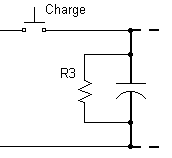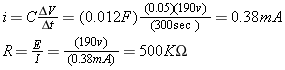# Bleeder Resistor

An electrolytic capacitor can store considerable charge for weeks and months. How do we ensure this does not endanger people?

Every high-power capacitor should have a bleeder resistor to remove a dangerous charge.

## Bleeder ResistorR3 is the safety bleeder resistor. It ensures the capacitor is drained during overnight storage to improve safety. We will examine long-term and short-term discharge rates to determine the appropriate resistance.

## Long-term Discharge:

If the charging time is 5 sec, then the bleed time should be at least 100x longer and preferably 1000x longer, or 83 minutes (e.g. 5,000 sec). Assume this is 3x the RC time constant, which then becomes a desired t = RC = 1600 seconds or longer.

If t = RC, then R3 = t/C = (1600 sec) / (0.012 F) = 133 Kohm

Now check the power used in the bleeder resistor.

P = V2/R = (200 v)2 / (133 Kohm) = 0.3 watts

Therefore a conservative rating would be 1-watt resistor or larger.

Some experimentation found that a 150 Kohm 1-watt carbon composition resistor showed no noticeable warming. However, a 47 Kohm 1-watt resistor became quite warm.

## Short-term Discharge

The operator needs some time with a reasonably stable capacitor voltage between charging it up and firing the coilgun.

Let’s assume we want to lose < 5% of the charge in five minutes. This permits plenty of time between charge and discharge.

For short periods at full charge, the decay is closely approximated by a linear rate.Based on the calculations for short-term (500 Kohm) and long-term (133 Kohm) values, and some experimentation, the coilgun used a 150 Kohm 1-watt resistor.

The smaller value was chosen because a little faster decay was useful when the capacitor was charged a few volts beyond a target value. If it received, say, 52v instead of 50v, then we need to wait only a short time for it to return to the desired voltage.

 < Previous Page 12 of 37 Next >